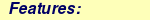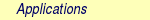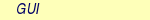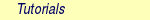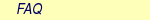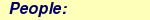MOLCAS manual:Next: 6.15 Other Multiconfigurational and Up: 6. Program Based Tutorials Previous: 6.13 CPF

Subsections

# 6.14 CCSDT -- A Set of Coupled-Cluster Programs

The MOLCAS program CCSDT computes Coupled-Cluster Singles Doubles, CCSD, and Coupled-Cluster Singles Doubles and Non-iterative Triples Correction CCSD(T) wave functions for restricted single reference both closed- and open-shell systems.

In addition to the ONEINT and ORDINT integral files (in non-Cholesky calculations), the CCSDT code requires the JOBIPH file containing the reference wave function (remember that it is not possible to compute open-shell systems with the SCF program) and the transformed two-electron integrals produced by the MOTRA module and stored in the TRAINT file.

Previously to execute the CCSDT module, wave functions and integrals have to be prepared. First, a RASSCF calculation has to be run in such a way that the resulting wave function has one single reference. In closed-shell situations this means to include all the orbitals as inactive and set the number of active electrons to zero. Keyword OUTOrbitals followed by the specification CANOnical must be used in the RASSCF input to activate the construction of canonical orbitals and the calculation of the CI-vectors on the basis of the canonical orbitals. After that the MOTRA module has to be run to transform the two-electron integrals using the molecular orbitals provided by the RASSCF module. The files JOBIPH or RASORB from the RASSCF calculation can be used directly by MOTRA using the keywords JOBIph or LUMOrb in the MOTRA input. Frozen or deleted orbitals can be introduced in the transformation step by the proper options in the MOTRA input.

## 6.14.1 CCSDT Outputs

The section of the MOLCAS output corresponding to the CC program is self explanatory. The default output simply contains the wave function specifications from the previous RASSCF calculation, the orbital specifications, the diagonal Fock matrix elements and orbital energies, the technical description of the calculation, the iterations leading to the CCSD energy, and the five largest amplitudes of each type, which will help to evaluate the calculation. If triples excitations have been required the description of the employed method (from the three available) to compute perturbatively the triple excited contributions to the CC energy, the value of the correction, and the energy decomposition into spin parts will be available.

## 6.14.2 Example of a CCSD(T) calculation

Figure 6.13 contains the input files required by the seward, scf, rasscf, motra and ccsdt programs to compute the ground state of the HF+ cation. molecule, which is a doublet ofsymmetry. A more detailed description of the different options included in the input of the programs can be found in the CCSDT section of the user's guide. This example describes how to calculate CCSD(T) energy for HF(+) cation. This cation can be safely represented by the single determinant as a reference function, so one can assume that CCSD(T) method will be suitable for its description.

The calculation can be divided into few steps:

1. Run SEWARD to generate AO integrals.
2. Calculate the HF molecule at the one electron level using SCF to prepare an estimate of MO for the RASSCF run.
3. Calculate HF(+) cation by subtracting one electron from the orbital with the first symmetry. There is only one electron in one active orbital so only one configuration is created. Hence, we obtain a simple single determinant ROHF reference.
4. Perform MO transformation exploiting MOTRA using MO coefficients from the RASSCF run.
5. Perform the Coupled Cluster calculation using CCSDT program. First, the data produced by the programs RASSCF and MOTRA need to be reorganized, then the CCSD calculation follows, with the chosen spin adaptation being T2 DDVV. Finally, the noniterative triple excitation contribution calculation is following, where the CCSD amplitudes are used.

This is an open shell case, so it is suitable to choose CCSD(T) method as it is defined by Watts et al. . Since CCSD amplitudes produced by previous CCSD run are partly spin adapted and denominators are produced from the corresponding diagonal Fock matrix elements, final energy is sometimes referred as SA1(see ).&SEWARD  &END
Title=  HF  molecule
Symmetry
X  Y
Basis  set
F.ANO-S-VDZ
F  0.00000  0.00000  1.73300
End  of  basis
Basis  set
H.ANO-S-VDZ
H  0.00000  0.00000  0.00000
End  of  basis
End  of  input
&SCF
&RASSCF
Title=  HF(+)  cation
OUTOrbitals=  Canonical
Symmetry=  1;  Spin=  2
nActEl=  1  0  0;  Inactive=  2  1  1  0;  Ras2=  1  0  0  0
LumOrb;  OUTOrbitals=  Canonical
&MOTRA;  JobIph;  Frozen=  1  0  0  0
&CCSDT
Iterations=  50;  Shift=  0.2,0.2;  Accuracy=  1.0d-7
Denominators=  2;  Extrapolation=  5,4
Adaptation=  1;  Triples=  3;  T3Denominators=  0

RASSCF calculates the HF ionized state by removing one electron from the orbital in the first symmetry. Do not forget to use keyword CANONICAL. In the CCSDT run, the number of iterations is limited to 50. Denominators will be formed using orbital energies. (This corresponds to the chosen spin adaptation.) Orbitals will be shifted by 0.2 au, what will accelerate the convergence. However, final energy will not be affected by the chosen type of denominators and orbital shifts. Required accuracy is 1.0d-7 au. for the energy. T2 DDVV class of CCSD amplitudes will be spin adapted. To accelerate the convergence, DIIS procedure is exploited. It will start after 5th iteration and the last four iterations will be taken into account in each extrapolation step.

In the triples step the CCSD(T) procedure as defined by Watts et al.  will be performed. Corresponding denominators will be produced using diagonal Fock matrix elements.

## 6.14.3 CCSDT - Basic and Most Common Keywords

 Keyword Meaning CCSD Coupled-cluster singles and doubles method CCT CCSD plus a non iterative triples (T) calculation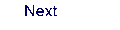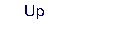Next: 6.15 Other Multiconfigurational and Up: 6. Program Based Tutorials Previous: 6.13 CPF